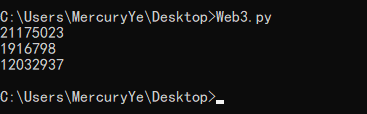# MD5截断比较验证from multiprocessing.dummy import Pool as tp
import hashlib

knownMd5 = '9331c'      #已知的md5明文def md5(text):
return hashlib.md5(str(text).encode('utf-8')).hexdigest()

def findCode(code):
key = code.split(':')
start = int(key)
end = int(key)
for code in range(start, end):
if md5(code)[0:5] == knownMd5:
print code
break
list=[]
for i in range(3):    #这里的range(number)指爆破出多少结果停止
list.append(str(10000000*i) + ':' + str(10000000*(i+1)))
pool = tp()    #使用多线程加快爆破速度
pool.map(findCode, list)
pool.close()
pool.join()posted @ 2019-08-04 11:08  Ye'sBlog  阅读(1081)  评论(0编辑  收藏